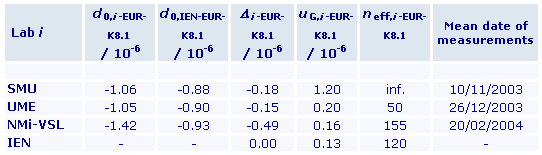Comparison
Comparison close
Results

CCEM.EM-K8, EUROMET.EM-K8, CCEM-K8.1 and EUROMET.EM-K8.1

MEASURAND : DC voltage ratio 1000 V / 10 V
NOMINAL VALUE : 100

Degrees of equivalence Di and expanded uncertainty Ui at a 95% level of confidence in 10-6

Click on the graph to get a closer view and the acronyms of the laboratories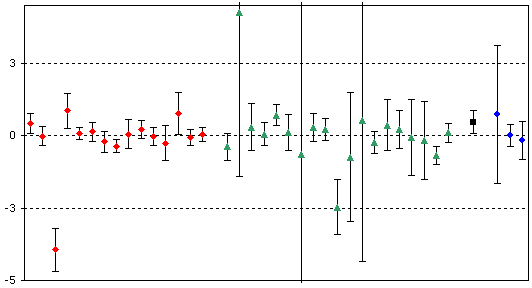Red diamonds: participants in CCEM-K8
Green triangles: participants in EUROMET.EM-K8
Black square: participant in CCEM-K8.1
Blue circles: participants in EUROMET.EM-K8.1

The dates of measurement are available from Laboratory Individual Meaurements.

The graph of equivalence can be printed out from page 8 of the .PDF file Summary Results.

CCEM.EM-K8, EUROMET.EM-K8, CCEM-K8.1 and EUROMET.EM-K8.1

MEASURAND : DC voltage ratio 100 V / 10 V
NOMINAL VALUE : 10

Degrees of equivalence Di and expanded uncertainty Ui at a 95% level of confidence in 10-6

Click on the graph to get a closer view and the acronyms of the laboratories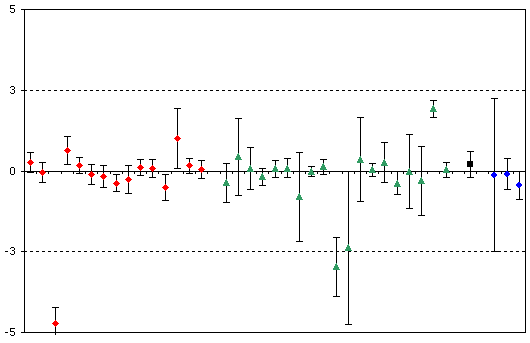Red diamonds: participants in CCEM-K8
Green triangles: participants in EUROMET.EM-K8
Black square: participant in CCEM-K8.1
Blue circles: participants in EUROMET.EM-K8.1

The dates of measurement are available from Laboratory Individual Meaurements.

The graph of equivalence can be printed out from page 16 of the .PDF file Summary Results.

Comparison
Comparison close
Results

CCEM-K8, EUROMET.EM-K8, CCEM-K8.1 and EUROMET.EM-K8.1

MEASURAND : DC voltage ratio 1000 V / 10 V
NOMINAL VALUE : 100

Degrees of equivalence Di and expanded uncertainty Ui at a 95% level of confidence:

• Participants in CCEM-K8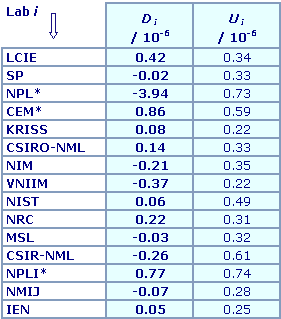* indicate the laboratories which did not participate in the computation of the
CCEM-K8 key comparison reference value

• Participants in EUROMET.EM-K8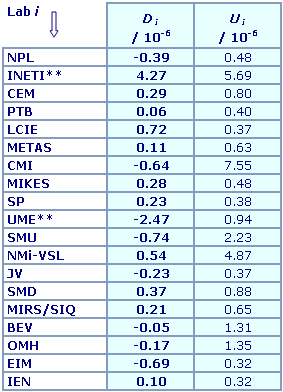** indicate the laboratories which did not participate in the computation of the EUROMET.EM-K8 reference value

• Participant in CCEM-K8.1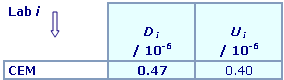• Participant in EUROMET.EM-K8.1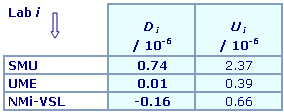The dates of measurement are available from Laboratory Individual Meaurements.

CCEM-K8, EUROMET.EM-K8, CCEM-K8.1 and EUROMET.EM-K8.1

MEASURAND : DC voltage ratio 100 V / 10 V
NOMINAL VALUE : 10

Degrees of equivalence Di and expanded uncertainty Ui at a 95% level of confidence:

• Participants in CCEM-K8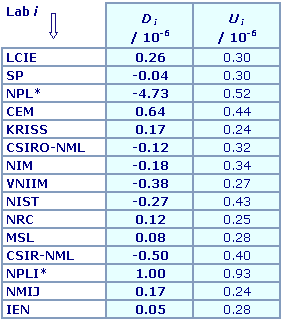* indicates the laboratories which did not participate in the computation of the
CCEM-K8 key comparison reference value

• Participants in EUROMET.EM-K8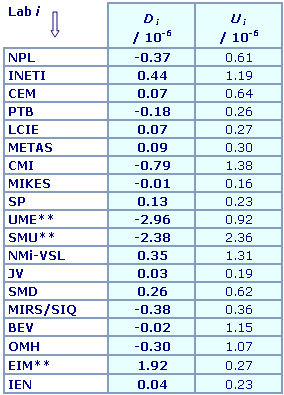** indicates the laboratories which did not participate in the computation of the EUROMET.EM-K8 reference value

• Participant in CCEM-K8.1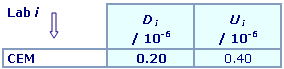• Participant in EUROMET.EM-K8.1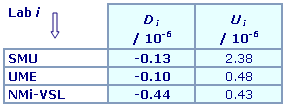The dates of measurement are available from Laboratory Individual Meaurements.

Comparison
Comparison close
CC comparison
 Metrology area, Sub-field Electricity and Magnetism, DC Voltage and Current Description Comparison of DC voltage ratios Time of measurements 1999 - 2001 Status Approved for equivalence
 Final Reports of the comparisons Measurand DC voltage ratio: 1000 V / 10 V and 100 V / 10 V Transfer device Datron 4902S standard divider, s/n 20335
 Comparison type Key Comparison Consultative Committee CCEM (Consultative Committee for Electricity and Magnetism) Conducted by CCEM (Consultative Committee for Electricity and Magnetism) Comments A subsequent comparison was decided between CEM, CSIR-NML and IEN. It is registered into the BIPM key comparison database under the identifier CCEM-K8.1.EUROMET.EM-K8 results, CCEM-K8.1 results, and EUROMET.EM-K8.1 results are linked to those of CCEM-K8. CCEM-K8 results were published on 16 January 2003.EUROMET.EM-K8 results were published on 16 January 2004, CCEM-K8.1 results on 03 June 2004, and EUROMET.EM-K8.1 results on 26 January 2005. Two DC voltage ratios, 1000 V / 10 V (nominal value: 100) and 100 V / 10 V (nominal value: 10) are measured using Datron standard dividers as travelling standards. Pilot institute IEN Istituto Elettrotecnico Nazionale Galileo Ferraris; from 1st January 2006: Istituto Nazionale di Ricerca Metrologica, INRIM Italy Contact person G. Marullo Reedtz +39 011 3919 421
First Name Last Name
wwww@ww.www +356719836 Institute 1 Institute 1 Khmelnitskiy
Pilot laboratory
IEN

Istituto Elettrotecnico Nazionale Galileo Ferraris; from 1st January 2006: Istituto Nazionale di Ricerca Metrologica, INRIM, Italy, EURAMET

 BNM-LCIE Bureau National de Métrologie - Laboratoire Central des Industries Electriques (became LNE in 2005), France, EURAMET
 CEM Centro Español de Metrologia, Spain, EURAMET
 CSIR-NML Council for Scientific and Industrial Research, National Metrology Laboratory (became NMISA in 2007), South Africa, AFRIMETS
 CSIRO-NML Commonwealth Scientific and Industrial Research Organization - National Measurement Laboratory; became National Measurement Institute, Australia, NMIA in 2004, Australia, APMP
 KRISS Korea Research Institute of Standards and Science, Korea, Republic of, APMP
 MSL Measurement Standards Laboratory, New Zealand, APMP
 NIM National Institute of Metrology, China, APMP
 NIST National Institute of Standards and Technology, United States, SIM
 NMIJ AIST National Metrology Institute of Japan, Japan, APMP
 NPL National Physical Laboratory, United Kingdom, EURAMET
 NPLI CSIR National Physical Laboratory of India, India, APMP
 NRC National Research Council, Canada, SIM
 SP Technical Research Institute of Sweden from 2017 Research Institutes of Sweden AB, Sweden, EURAMET
 VNIIM D.I. Mendeleyev Institute for Metrology, Rosstandart, Russian Federation, COOMET
Comparison
Comparison close
Results

CCEM-K8, EUROMET.EM-K8, CCEM-K8.1 and EUROMET.EM-K8.1

MEASURAND : DC voltage ratio 1000 V / 10 V
NOMINAL VALUE : 100

• Key comparison CCEM-K8

 The key comparison reference value,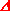R, is the arithmetic mean of the differencesi, obtained from twelve participants. (Statistical tests indicate that there is a high probability that the result of each of the other three participants does not belong to the same statistical distribution as the other twelve).The mean relative deviation of the ratio from nominal is given by the sumR + d0,IEN. The standard uncertainty, u(R), associated withR is the standard deviation of the mean.R = -0.048x10-6 with standard uncertainty of 0.062x10-6
and 11 degrees of freedom

 The degree of equivalence of each laboratory with respect to the reference value is given by a pair of terms: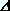Di = (i - R), and the corresponding expanded uncertainty Ui, assessed for a level of confidence of 95%. For the 12 laboratories contributing to the definition of the reference value, the correlation with the reference value is taken into account in the computation of Ui.
• Key comparison EUROMET.EM-K8

 The reference value of EUROMET.EM-K8,R-EUR, is the arithmetic mean of the differencesi-EUR, obtained from seventeen participants. (Statistical tests indicate that there is a high probability that the result of each of the two other participants does not belong to the same statistical distribution as the other seventeen).The mean relative deviation of the ratio from nominal is given by the sumR-EUR + d0,IEN-EUR. The standard uncertainty associated withR-EUR is the standard deviation of the mean.R-EUR = -0.097x10-6 with standard uncertainty of 0.103x10-6
and 16 degrees of freedom

 The degree of equivalence of each laboratory with respect to the reference value is given by a pair of terms:Di-EUR = (i-EUR - R-EUR), and the corresponding expanded uncertainty Ui-EUR, assessed for a level of confidence of 95%. For the 17 laboratories contributing to the definition of the reference value, the correlation with the reference value is taken into account in the computation of Ui-EUR.

In a measurement of voltage ratio, no national standard is involved. Therefore any participant in EUROMET.EM-K8 and CCEM-K8 comparisons can be considered equally able, in principle, to perform an unbiased measurement of the travelling standard. Since the number of participants in each comparison is significant and different methods of measurement were used, it is unlikely that the corresponding comparison reference values are affected by systematic errors. Therefore they are equivalent and the degrees of equivalence obtained from the EUROMET.EM-K8 comparison are assumed to be the same as if the participants had taken part in the CCEM-K8 comparison:
Di = Di-EUR and Ui = Ui-EUR for the participants in EUROMET.EM-K8 comparison.

The Linkage Report gives a verification of this assumption based on the results of the common participants in both comparisons.

 Because voltage ratios, and not national standards, were the object of these comparisons, the CCEM has decided not to publish the table of the bilateral degrees of equivalence, which are not really significant in the present case. They can, however, be calculated by the difference of the Di values, with corresponding 95% uncertainty given, within an approximation of about 5% or better, by twice the root-sum-square of the global standard uncertainties of the two laboratories involved.

 The degree of equivalence Di of laboratory i with respect to the key comparison reference valueR, is given by the estimated difference(i - R) that the laboratory would have obtained if it had directly participated in comparison CCEM-K8, and by the corresponding 95% expanded uncertainty Ui. The link to CCEM-K8 is given by IEN, who was the pilot laboratory in both comparisons, with:Di = (i - IEN)CCEM-K8.1 + (IEN - R)CCEM-K8 = (i-K8.1 -IEN-K8.1) + DIENui = (u2G,i-K8.1 + u2transfer + u2(R))1/2,where utransfer represents the standard uncertainty of the link to CCEM-K8, evaluated to 0.08x10-6. The degrees of freedom are taken into account in the calculation of Ui.

 The link between EUROMET.EM-K8.1 and CCEM-K8 is computed in the same way, with utransfer = 0.08x10-6. The degrees of freedom have negligible influence in the calculation of Ui.

 The bilateral degrees of equivalence of laboratory i having participated in CCEM-K8.1 or EUROMET.EM-K8.1, with respect to any other laboratory participating in CCEM-K8 or EUROMET.EM-K8, can be calculated by the difference of the Di values of the two laboratories, with corresponding 95% uncertainty given, within an approximation of 5% or better, by twice the root-sum-square of three terms: the global standard uncertainty of laboratory i, the transfer standard uncertainty utransfer and the global standard uncertainty of the other laboratory.

CCEM-K8, EUROMET.EM-K8, CCEM-K8.1 and EUROMET.EM-K8.1

MEASURAND : DC voltage ratio 100 V / 10 V
NOMINAL VALUE : 10

• Key comparison CCEM-K8

 The key comparison reference value,R, is the arithmetic mean of the differencesi, obtained from thirteen participants. (Statistical tests indicate that there is a high probability that the result of each of the other two participants does not belong to the same statistical distribution as the other thirteen).The mean relative deviation of the ratio from nominal is given by the sumR + d0,IEN. The standard uncertainty, u(R), associated withR is the standard deviation of the mean.R = -0.050x10-6 with standard uncertainty of 0.083x10-6
and 12 degrees of freedom

 The degree of equivalence of each laboratory with respect to the reference value is given by a pair of terms:Di = (i - R), and the corresponding expanded uncertainty Ui, assessed for a level of confidence of 95%. For the 13 laboratories contributing to the definition of the reference value, the correlation with the reference value is taken into account in the computation of Ui.
• Key comparison EUROMET.EM-K8

 The reference value of EUROMET.EM-K8,R-EUR, is the weighted mean of the differencesi-EUR, obtained from sixteen participants. (Statistical tests indicate that there is a high probability that the result of each of the three other participants does not belong to the same statistical distribution as the other sixteen).The mean relative deviation of the ratio from nominal is given by the sumR-EUR + d0,IEN-EUR. The standard uncertainty associated withR-EUR is the weighted standard uncertainty.R-EUR = -0.039x10-6 with standard uncertainty of 0.039x10-6
and 105 degrees of freedom

 The degree of equivalence of each laboratory with respect to the reference value is given by a pair of terms:Di-EUR = (i-EUR - R-EUR), and the corresponding expanded uncertainty Ui-EUR, assessed for a level of confidence of 95%. For the 16 laboratories contributing to the definition of the reference value, the correlation with the reference value is taken into account in the computation of Ui-EUR.

In a measurement of voltage ratio, no national standard is involved. Therefore any participant in EUROMET.EM-K8 and CCEM-K8 comparisons can be considered equally able, in principle, to perform an unbiased measurement of the travelling standard. Since the number of participants in each comparison is significant and different methods of measurement were used, it is unlikely that the corresponding comparison reference values are affected by systematic errors. Therefore they are equivalent and the degrees of equivalence obtained from the EUROMET.EM-K8 comparison are assumed to be the same as if the participants had taken part in the CCEM-K8 comparison:
Di = Di-EUR and Ui = Ui-EUR for the participants in EUROMET.EM-K8 comparison.

The Linkage Report gives a verification of this assumption based on the results of the common participants in both comparisons.

 Because voltage ratios, and not national standards, were the object of these comparisons, the CCEM has decided not to publish the table of the bilateral degrees of equivalence, which are not really significant in the present case. They can, however, be calculated by the difference of the Di values, with corresponding 95% uncertainty given, within an approximation of about 10% or better, by twice the root-sum-square of the global standard uncertainties of the two laboratories involved.

 The degree of equivalence Di of laboratory i with respect to the key comparison reference valueR, is given by the estimated difference(i - R) that the laboratory would have obtained if it had directly participated in comparison CCEM-K8, and by the corresponding 95% expanded uncertainty Ui. The link to CCEM-K8 is given by IEN, who was the pilot laboratory in both comparisons. It is given by:Di = (i - IEN)CCEM-K8.1 + (IEN - R)CCEM-K8 = (i-K8.1 -IEN-K8.1) + DIENui = (u2G,i-K8.1 + u2transfer + u2(R))1/2,where utransfer represents the standard uncertainty of the link to CCEM-K8, evaluated to utransfer = 0.12x10-6. The degrees of freedom are taken into account in the calculation of Ui.

 The link between EUROMET.EM-K8.1 and CCEM-K8 is computed in the same way, with utransfer = 0.12x10-6. The degrees of freedom have negligible influence in the calculation of Ui.

 The bilateral degrees of equivalence of laboratory i having participated in CCEM-K8.1 or EUROMET.EM-K8.1, with respect to any other laboratory participating in CCEM-K8 or EUROMET.EM-K8, can be calculated by the difference of the Di values of the two laboratories, with corresponding 95% uncertainty given, within an approximation of 5% or better, by twice the root-sum-square of three terms: the global standard uncertainty of laboratory i, the transfer standard uncertainty utransfer and the global standard uncertainty of the other laboratory.
Comparison
Comparison close
Results

CCEM-K8, EUROMET.EM-K8, CCEM-K8.1 and EUROMET.EM-K8.1

MEASURAND : DC voltage ratio 1000 V / 10 V
NOMINAL VALUE : 100
Pilot laboratory: IEN

d0,i: fractional difference from nominal value of ratio x0,i, measured by laboratory i and corrected to standard ambient conditions; it is given by: x0,i = 100 x (1 + d0,i)
The fractional differences d0,IEN assigned by IEN to the ratio are obtained by interpolation of the IEN measurement results to the measurement dates of the other laboratories.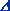i = (d0,i - d0,IEN)
uG,i: global standard uncertainty of laboratory i
neff,i: number of effective degrees of freedom of laboratory i

Note: the additional indexes "-K8.1", "-EUR" and "-EUR-K8.1" are used for the CCEM-K8.1, EUROMET.EM-K8 and EUROMET.EM-K8.1 key comparisons.

• Key comparison CCEM-K8
TRAVELLING STANDARD : voltage divider Datron 4902S, s/n 20335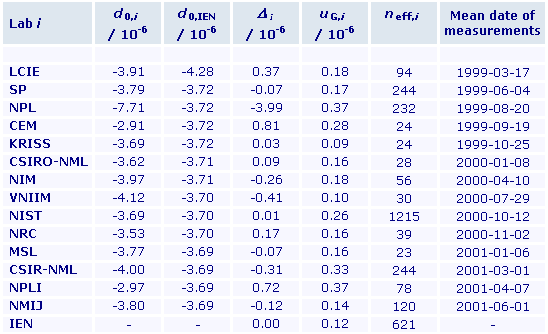• Key comparison EUROMET.EM-K8
TRAVELLING STANDARD : voltage divider Datron 4902S, s/n 12422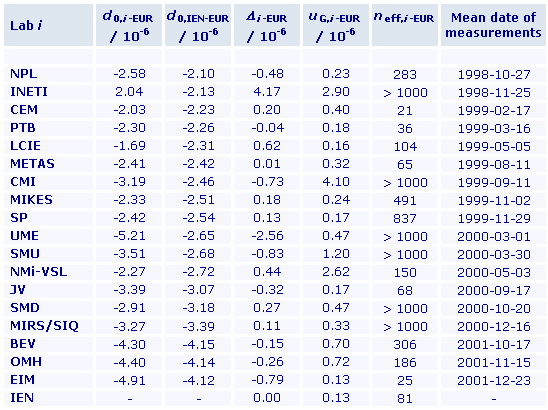• Key comparison CCEM-K8.1
TRAVELLING STANDARD : voltage divider Datron 4902S, s/n 20335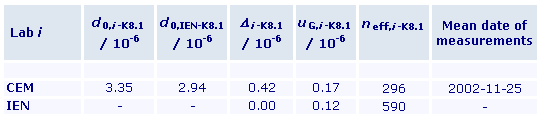• Key comparison EUROMET.EM-K8.1
TRAVELLING STANDARD : voltage divider Datron 4902S, s/n 20335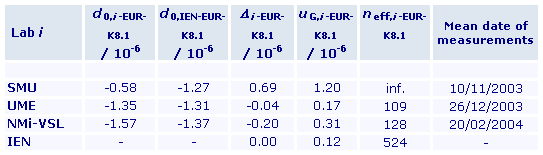CCEM-K8, EUROMET.EM-K8, CCEM-K8.1 and EUROMET.EM-K8.1

MEASURAND : DC voltage ratio 100 V / 10 V
NOMINAL VALUE : 10
Pilot laboratory: IEN

d0,i: fractional difference from nominal value of ratio x0,i, measured by laboratory i and corrected to standard ambient conditions; it is given by: x0,i = 10 x (1 + d0,i)
The fractional differences d0,IEN assigned by IEN to the ratio are obtained by interpolation of the IEN measurement results to the measurement dates of the other laboratories.i = (d0,i - d0,IEN)
uG,i: global standard uncertainty of laboratory i
neff,i: number of effective degrees of freedom of laboratory i

Note : the additional indexes "-K8.1", "-EUR" and "-EUR-K8.1" are used for the CCEM-K8.1, EUROMET.EM-K8 and EUROMET.EM-K8.1 key comparisons.

• Key comparison CCEM-K8
TRAVELLING STANDARD : voltage divider Datron 4902S, s/n 20335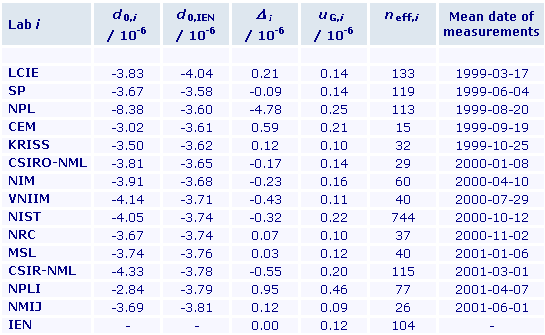• Key comparison EUROMET.EM-K8
TRAVELLING STANDARD : voltage divider Datron 4902S, s/n 12422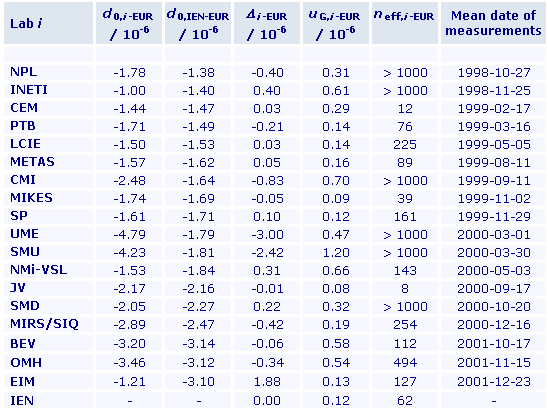• Key comparison CCEM-K8.1
TRAVELLING STANDARD : voltage divider Datron 4902S, s/n 20335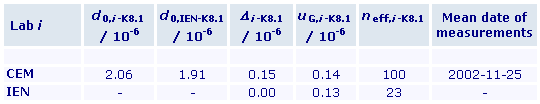• Key comparison EUROMET.EM-K8.1
TRAVELLING STANDARD : voltage divider Datron 4902S, s/n 20335